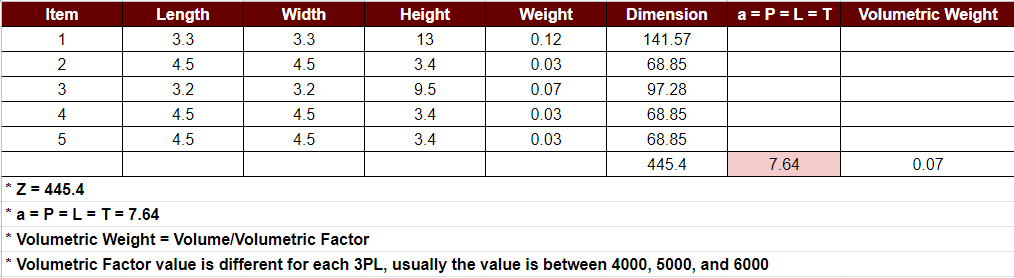# Weight Calculation

## Weight Calculation

The final weight of an Order will be selected from the heaviest weight between actual weight and volumetric weight. Every logistic and service have their own volumetric weight formula (volumetric divided by 4000 or 5000 or 6000).

The final weight of an Order must be calculated from items that have been packed, if there are multiple items, we assume that all items have been packaged into one package.

## Dimension Calculation with Multiple Items

For multiple items calculation and you don't have the standardized package. You can use this calculation to help you calculate the estimate length, width, and height to be use in the Pricing API and Create Order API.

The calculation is:

(P x L x T) + (P x L x T) = Z
Then square root of 3 the Z value = a
Then, you can input the 'a' value in L, W, H
P = L = T = a

P = Length
L = Width
T = Height
Z = Total Dimension